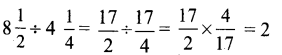# Samacheer Kalvi 6th Maths Solutions Term 3 Chapter 1 Fractions Intext Questions## Tamilnadu Samacheer Kalvi 6th Maths Solutions Term 3 Chapter 1 Fractions Intext Questions

(Try These Textbook Page No. 2)

Question 1.
If all the three cakes are divided among the total participants of the function what would be each one’s share? Discuss.
Solution:
Total participants of the function = 9
Total number of cakes = 3
∴ Each cake should be divided into 3 equal parts.
∴ Total number of equal parts of cake = 9
∴ Each one’s share may be $$\frac{1}{9}$$ of total cakes or $$\frac{1}{3}$$ of a cake.

Question 2.
Observe the following and represent the shaded parts as fraction.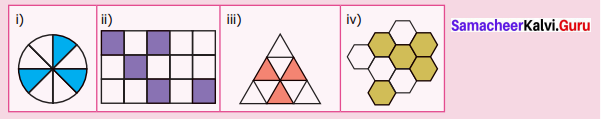Solution:
(i) Total number of equal parts = 8
Fraction representing the shaded parts = $$\frac{3}{8}$$

(ii) Total number of equal parts = 15
∴ Fraction representing the shaded parts = $$\frac{5}{15}$$

(iii) Total number of equal parts = 9
∴ Fraction representing the shaded parts = $$\frac{3}{9}$$

(iv) Total number of equal parts = 9
Fraction representing the shaded parts = $$\frac{5}{9}$$

Question 3.
Look at the following beakers, express the quantity of water as fraction and arrange them in ascending order: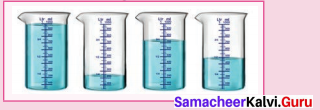Solution:
Quantity of water in the first beaker = 1 full
Quantity of water in the second beaker = $$\frac{1}{4}$$
Quantity of water in the third beaker = $$\frac{3}{4}$$
Quantity of water in the fourth beaker = $$\frac{1}{2}$$
Ascending order $$\frac{1}{4}$$ < $$\frac{1}{2}$$ < $$\frac{3}{4}$$ < 1

Question 4.
Write the fraction of shaded part in the following.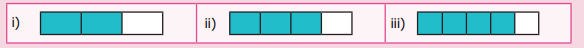Solution:
(i) Total number of equal parts = 3
Fraction representing the shaded portion = $$\frac{2}{3}$$

(ii) Total number of equal parts = 4
Fraction representing the shaded parts = $$\frac{3}{4}$$

(iii) Total number of equal parts = 5
Fraction representing the shaded parts = $$\frac{4}{5}$$Question 5.
Write the fraction that represents the dots In the triangle.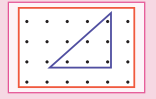Solution:
Total number of dots = 24
Number of dots in the triangle = 6
∴ Fraction represents the dots in the triangle = $$\frac{6}{24}$$

Question 6.
Find the fractions of the shaded and unshaded portions in the following.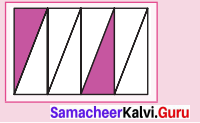Solution:
(a) Total number of equal parts = 8
∴ Fraction representing shaded parts = $$\frac{2}{8}$$

(b) Total number of equal parts = 8
∴ Fraction of unshaded portion = $$\frac{6}{8}$$

(Activity Textbook Page No. 3)

Take a rectangular paper. Fold it into two equal parts. Shade one part, write the fraction. Again fold it into two halves. Write the fraction for the shaded part. Continue this process 5 times and write the fraction of the shaded part. Establish the equivalent fractions of $$\frac{1}{2}$$ in the folded paper to your friends.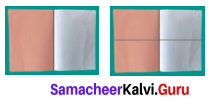Solution:
First time = $$\frac{1}{2}$$
Second time = $$\frac{2}{4}$$
Third time = $$\frac{4}{8}$$
Fourth time = $$\frac{8}{16}$$
Fifth time = $$\frac{16}{32}$$(Try These Textbook Page No. 4)

Question 1.
Find the unknown in the following equivalent fractions.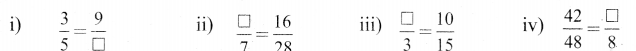Solution: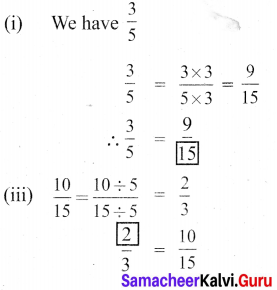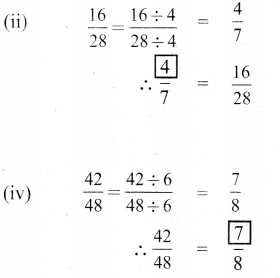(Try These Textbook Page No. 7)

Question 1.
Shade the rectangle for the given pair of fractions and say which is greater among them.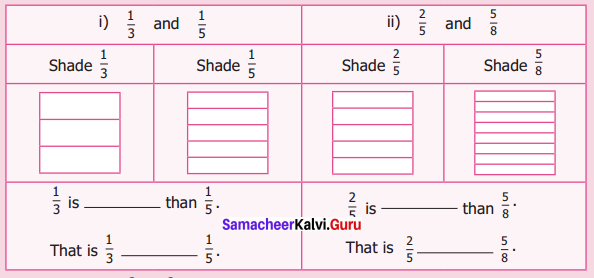Solution: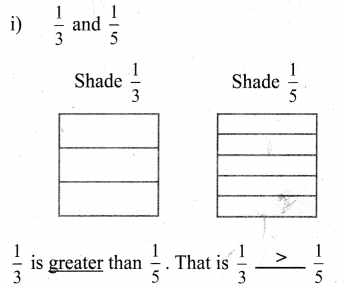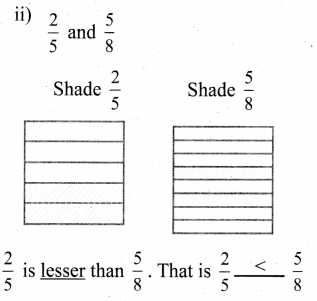Question 2.
Which is greater $$\frac{3}{8}$$ or $$\frac{3}{5}$$ ?
Solution:
LCM of the denominators 8 and 5 is 40.
Finding the equivalent fractions.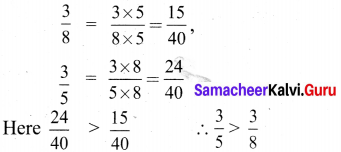Question 3.
Arrange the fractions in ascending order : $$\frac{3}{5}, \frac{9}{10}, \frac{11}{15}$$
Solution: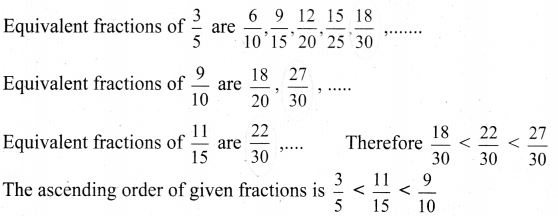Question 4.
Arrange the fractions in descending order : $$\frac{9}{20}, \frac{3}{4}, \frac{7}{12}$$
Solution: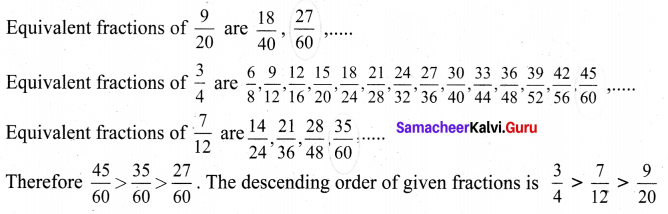(Try These Textbook Page No. 9)

(i) $$\frac{2}{3}+\frac{5}{7}$$
Solution:
By cross multiplication technique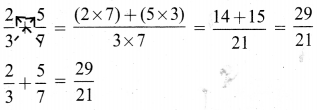(ii) $$\frac{3}{5}-\frac{3}{8}$$
Solution:
By cross multiplication technique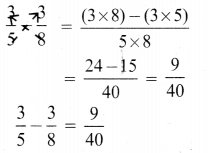(Activity Textbook Page No. 10)

Question 1.
Using the given fractions $$\frac{1}{5}, \frac{1}{6}, \frac{1}{10}, \frac{1}{15}, \frac{2}{15}, \frac{4}{15}, \frac{1}{30}, \frac{7}{30} \text { and } \frac{9}{30}$$ fill in the missing ones in the given 3 × 3 square in such a way that the addition of fractions through rows, columns and diagonals give the same total $$\frac{1}{2}$$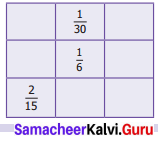Solution: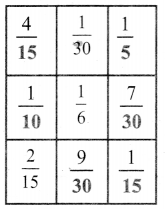(Try These Textbook Page No. 10)

Question 1.
Complete the following table. The first one is done for you.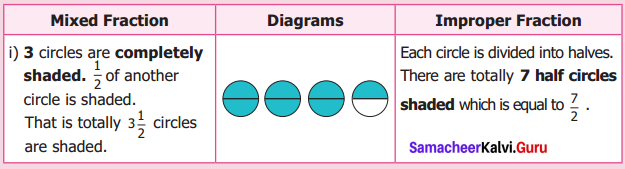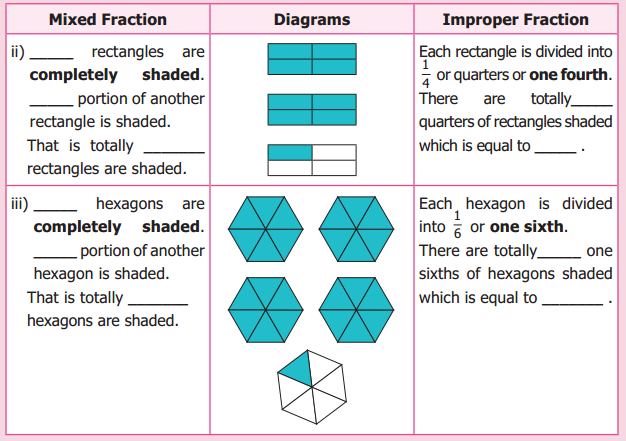(Try These Textbook Page No. 11)

Question 1.
(i) Are 5$$\frac{2}{3}$$ and 5$$\frac{4}{6}$$ equal?
Solution: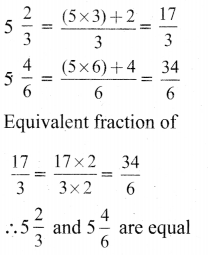(ii) $$\frac{3}{2} \neq 3 \frac{1}{2}$$ why?
Solution: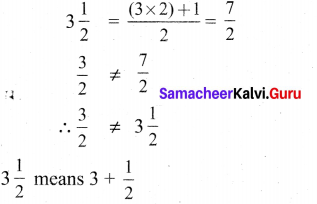Question 2.
Convert 3$$\frac{1}{3}$$ into improper fraction.
Solution: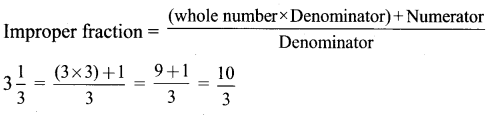Question 3.
Convert $$\frac{45}{7}$$ into mixed fraction.
Solution: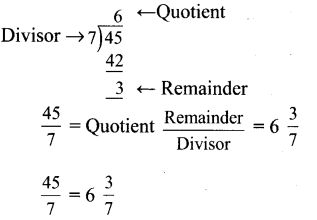(Try These Textbook Page No. 13)

Question 1.
Find the sum of 5$$\frac{4}{9}$$ and 3$$\frac{1}{6}$$.
Solution: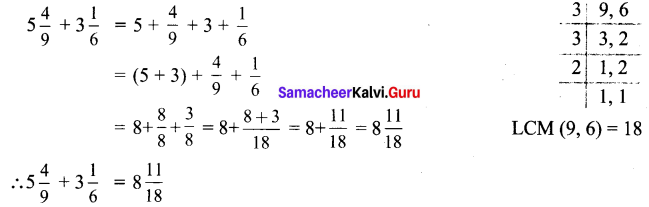Question 2.
Subtract 7$$\frac{1}{6}$$ and 12$$\frac{3}{8}$$
Solution: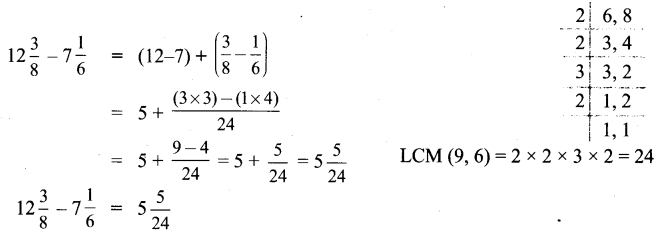Question 3.
Subtract the sum of 6$$\frac{1}{6}$$ and 3$$\frac{1}{5}$$ from the sum of 9$$\frac{2}{3}$$ and 2$$\frac{1}{2}$$.
Solution: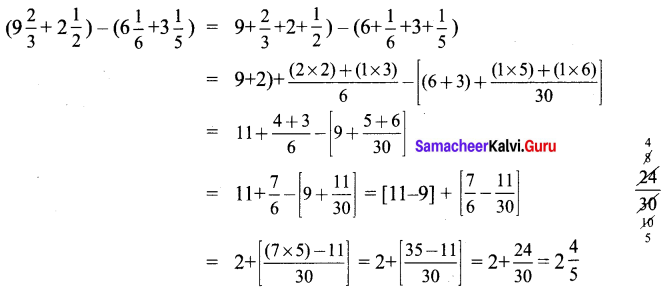(Try These Textbook Page No. 15)

Question 1.
2$$\frac{1}{4}$$ × 3 is not equal to 6$$\frac{1}{4}$$. Why?
Solution: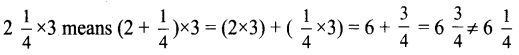Question 2.
Simplify : 35 × $$\frac{3}{7}$$.
Solution: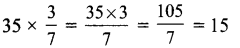Question 3.
Find the value of $$\frac{1}{5}$$ of 15.
Solution:
$$\frac{1}{5}$$ of 15 = $$\frac{1}{5}$$ × 15 = $$\frac{15}{5}$$ = 3

Question 4.
Find the value of $$\frac{1}{3}$$ of $$\frac{3}{4}$$
Solution: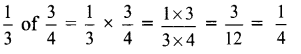Question 5.
Multiply 7$$\frac{3}{4}$$ by 5$$\frac{1}{2}$$.
Solution: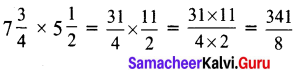(Activity Textbook Page No. 15)

Take a paper. Fold it into 4 parts vertically of equal width. Shade one part of it with red. Then, fold it into 3 parts horizontally of equal width. Shade two parts of it with blue. Now, you count the number of shaded grids which have both the colours. (Hint: The total number of grids is the product of $$\frac{2}{3}$$ and $$\frac{1}{4}$$)
Solution:
Activity to be done by the students themselves

(Try These Textbook Page No. 17)

Question 1.
(i) How many 6s are there in 18?
Solution:
Number of 6s in 18 are $$\frac{18}{6}$$ = 3

(ii) How many $$\frac{1}{4}$$s are there in 5?
Solution:
Number of $$\frac{1}{4}$$ s in 5 are 5 ÷ $$\frac{1}{4}$$ = 5 × $$\frac{4}{1}$$ = 20

(iii) $$\frac{1}{3}$$ ÷ 5 = ?
Solution:
$$\frac{1}{3}$$ ÷ 5 = $$\frac{1}{3} \times \frac{1}{5}$$ = $$\frac{1}{15}$$

(Try These Textbook Page No. 18)

Question (i)
Find the value of 5 ÷ 2$$\frac{1}{2}$$.
Solution: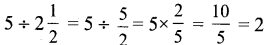Question (ii)
Simplify: $$1 \frac{1}{2} \div \frac{1}{2}$$
Solution: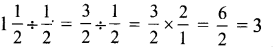Question (iii)
Divide 8 $$\frac{1}{2}$$ by 4$$\frac{1}{4}$$.
Solution: